## show/hide words to know

Area: used to describe the surface space of an object. Example: the floor area of your room is 100 square feet if it measures 10 feet by 10 feet......more

Magnify: to increase the size of something. Making an object larger by using a tool or instrument such as a magnifying glass or microscope.

Simulate: to look like, take the shape of, or sound like something else; imitate.

## Scale Activity Part 1

The following activity was presented in the "Tiny Matter" Ask A Biologist Podcast. These are the steps we took to see how big a one inch line would become if it were to be magnified one hundred thousand times and then a billion times. After you follow the activity, try out your math skills with an activity we have outlined at the end.

 It is difficult to think about tiny things we cannot see. Let's take something we can see and magnify it several times to see the results. Grab a pencil, a piece of paper, and a ruler. Next draw a line one inch long.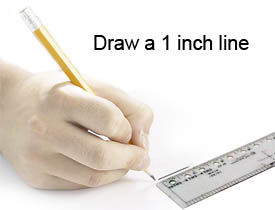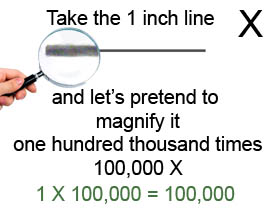Using our imagination and some mathematics we are going to magnify the line 100,000 times. You can see from the use of multiplication, that we have a line that is 100,000 inches long. How long is 100,000 inches? First let's see how many feet 100,000 inches is by dividing 100,000 by 12 (the number of inches in a foot). The result of our calculation shows that the line is 8,333 feet long.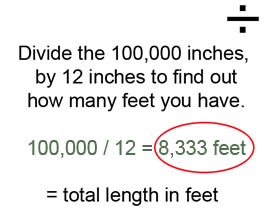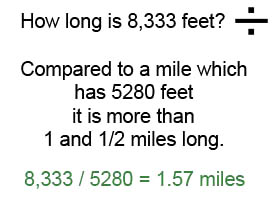To help understand just how long 8,333 feet is we can divide the total number of feet by the number of feet in a mile (5280). Now we can see the one inch line we just drew is now over 1 1/2 miles long! So far the length of the magnified line has been calculated. How about the width of the line? Depending on the size of the tip of your pencil, the width will vary. The line we drew was 1/36 of an inch wide. If we divide the length of the magnified line by 36 we will know the width of the line.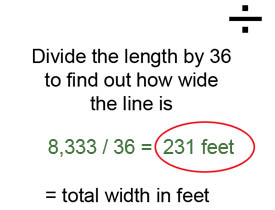Really, how big is this line? All these calculations give us an idea of how large the line can get when we magnifying it 100,000 times, but it still does not give an idea of how really large the line is. So we measured the length and width of a bright yellow Volkswagen Beetle to see how many we could place on the magnified line.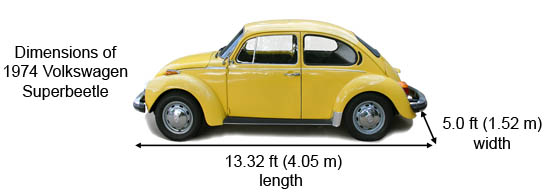If we could line up all the bright yellow Volkswagen Beetle cars on the line end-to-end, we would need 625 of the cars to equal the length of the line. If we could place all the bright yellow Volkswagen Beetle cars side-by-side across the width of the line, we would need 46 of the cars. How many cars could we line up end-to-end and across the magnified line?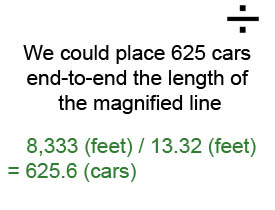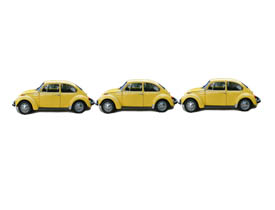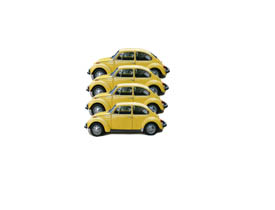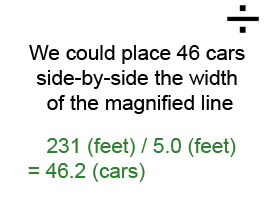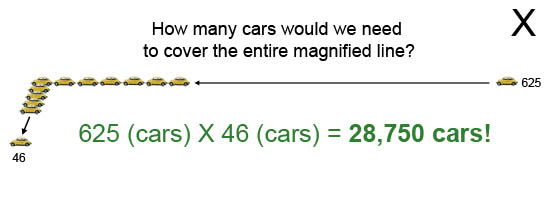What would happen if we magnified the line a billion times?Click to find out

Nanogear image from Wikimedia - Jie Han, Al Globus, Richard Jaffe and Glenn Deardorff.

## View Citation

You may need to edit author's name to meet the style formats, which are in most cases "Last name, First name."

## Bibliographic details:

• Article: Scale Activity Part 1
• Author(s): CJ Kazilek
• Publisher: Arizona State University School of Life Sciences Ask A Biologist
• Site name: ASU - Ask A Biologist
• Date published: November 2, 2009
• Date accessed: November 28, 2020

## APA Style

CJ Kazilek. (2009, November 02). Scale Activity Part 1. ASU - Ask A Biologist. Retrieved November 28, 2020 from https://askabiologist.asu.edu/fr/scale-activity

## Chicago Manual of Style

CJ Kazilek. "Scale Activity Part 1". ASU - Ask A Biologist. 02 November, 2009. https://askabiologist.asu.edu/fr/scale-activity

## MLA 2017 Style

CJ Kazilek. "Scale Activity Part 1". ASU - Ask A Biologist. 02 Nov 2009. ASU - Ask A Biologist, Web. 28 Nov 2020. https://askabiologist.asu.edu/fr/scale-activity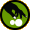Contribute